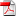## Lectures

After each lecture, you can watch the videos here.

Lecture 1: Introduction.Lecture 2: Classification.Lecture 3: Maximum likelihood.Lecture 4: Linear regression.Lecture 5: Optimization: Gradient descent, line search, stochastic gradient descent for massive datasets and streaming data.Lecture 6: Second order methods: Newton, L-BFGS, and iterative reweighted least squares.Lecture 7: Constrained optimization: Lagrangians and duality. Application to penalized maximum likelihood and Lasso.Lecture 8: Bayesian learning: Priors, posterior, predictive distributions, conjugate models, and cross-validation Vs marginal likelihood.Lecture 9: Multivariate Gaussian models.Lecture 10: Gaussian processes.Lecture 11: Directed probabilistic graphical models.Lecture 12: Undirected probabilistic graphical models, random fields, CRFs, deep learning and log-linear models.Lecture 13: Monte Carlo methods.Lecture 14: The EM algorithm, mixtures and clustering.## LATEST :

• Classes begin on January 11th.
• The machine learning book of Hastie, Tibshirani and Friedman is a good online alternative: The elements of statistical learning.
• The following handout should help you with linear algebra revision: PDF